Are you searching for the Samacheer Kalvi 12th Chemistry Chapter Wise Solutions PDF? Then, get your Samacheer Kalvi 12th Chapter Wise Solutions PDF for free on our website. Students can Download Chemistry Chapter 3 p-Block Elements – II Questions and Answers, Notes Pdf, Samacheer Kalvi 12th Chemistry Solutions Guide Pdf helps you to revise the complete Tamilnadu State Board New Syllabus and score more marks in your examinations.

## Tamilnadu Samacheer Kalvi 12th Chemistry Solutions Chapter 3 p-Block Elements – II

All concepts are explained in an easy way on our website. So, students can easily learn and practice Tamilnadu State Board 12th Chemistry Chapter 3 p-Block Elements – II Question and Answers. You can enjoy the digitized learning with the help of the Tamilnadu State Board Chemistry Online Material.

### Samacheer Kalvi 12th Chemistry p-Block Elements – II TextBook Evalution

I. Choose the correct answer.

12th Chemistry Chapter 3 Book Back Answers Question 1.
In which of the following, NH3 is not used?
(a) Nessler’s reagent
(b) Reagent for the analysis of IV group basic radical
(c) Reagent for the analysis of III group basic radical
(d) Tollen’s reagent
Answer:
(a) Nessler’s reagent

Samacheer Kalvi Guru 12th Chemistry Question 2.
Which is time regarding nitrogen?
(a) least electronegative element
(b) has low ionisation enthalpy than oxygen
(c) d-orbitals available
(d) ability to form pπ – pπ bonds with itself
Answer:
(d) ability to form pπ – pπ bonds with itself

12th Chemistry 3rd Lesson Book Back Answers Question 3.
An element belongs to group 15 and 3 rd period of the periodic table, its electronic configuration would be …………
(a) 1s22s22p4
(b) 1s22s22p3
(c) 1s22s22p63s23p2
(d) 1s22s22p63s23p3
Answer:
(d) 1s22s22p63s23p3

Samacheerkalvi.Guru 12th Chemistry Question 4.
Solid (A) reacts with strong aqueous NaOH liberating a foul smelling gas(B) which spontaneously bum in air giving smoky rings. A and B are respectively …………
(a) P4(red) and PH3
(b) P4(white) and PH3
(c) S8 and H2S
(d) P4(white) and H2S
Answer:
(b) P4(white) and PH3

Samacheer Kalvi Guru Class 12 Chemistry Question 5.
In the brown ring test, brown colour of the ring is due to …………
(a) a mixture of NO and NO2
(b) Nitroso ferrous sulphate
(c) Ferrous nitrate
(d) Ferric nitrate
Answer:
(b) Nitroso ferrous sulphate

Samacheer Kalvi 12th Chemistry Question 6.
On hydrolysis, PCl3 gives …………
(a) H3PO3
(b) PH3
(c) H3PO4
(d) POOL
Answer:
(a) H3PO3

Chemistry Class 12 Samacheer Kalvi Question 7.
P4O6 reacts with cold water to give …………
(a) H3PO3
(b) H4P2O7
(c) HPO3
(d) H3PO4
Answer:
(a) H3PO3

Samacheer Kalvi Class 12 Chemistry Solutions Question 8.
The basicity of pyrophosphorous acid ( H4P2O5) is …………
(a) 4
(b) 2
(c) 3
(d) 5
Answer:
(b) 2

Samacheer Kalvi Guru Chemistry Question 9.
The molarity of given orthophosphoric acid solution is 2M. its normality is …………
(a) 6N
(b) 4N
(c) 2N
(d) none of these
Answer:
(a) 6N

Chapter 3 Chemistry Class 12 Notes Question 10.
Assertion – bond dissociation energy of fluorine is greater than chlorine gas
Reason – chlorine has more electronic repulsion than fluorine
(a) Both assertion and reason are true and reason is the correct explanation of assertion.
(b) Both assertion and reason are true but reason is not the correct explanation of assertion.
(c) Assertion is true but reason is false.
(d) Both assertion and reason are false.
Answer:
(d) Both assertion and reason are false. The converse is true.

Samacheer Kalvi 12th Chemistry Solutions Question 11.
Among the following, which is the strongest oxidizing agent?
(a) Cl2
(b) F2
(c) Br2
(d) I2
Answer:
(b) F2

Class 12 Chemistry Samacheer Kalvi Question 12.
The correct order of the thermal stability of hydrogen halide is …………
(a) HI > HBr > HCl > HF
(b) HF > HCl > HBr > HI
(c) HCl > HF > HBr > HI
(d) HI > HCl > HF > HBr
Answer:
(b) HF > HCl > HBr > HI

Samacheer Kalvi 12 Chemistry Solutions Question 13.
Which one of the following compounds is not formed?
(a) XeOF4
(b) XeO3
(c) XeF2
(d) NeF2
Answer:
(d) NeF2

Class 12 Chemistry Chapter 3 Notes Pdf Download Question 14.
Most easily liquefiable gas is …………
(a) Ar
(b) Ne
(c) He
(d) Kr
Answer:
(c) He

12th Chemistry Chapter 7 Book Back Answers Question 15.
XeF6 on complete hydrolysis produces …………
(a) XeOF4
(b) XeO2F4
(c) XeO3
(d) XeO2
Answer:
(c) XeO3

Question 16.
On oxidation with iodine, sulphite ion is transformed to …………
(a) S4$${ O }_{ 6 }^{ 2- }$$
(b) S2$${ O }_{ 6 }^{ 2- }$$
(c) S$${ O }_{ 4 }^{ 2- }$$
(d) S$${ O }_{ 3 }^{ 2- }$$
Answer:
(c) S$${ O }_{ 4 }^{ 2- }$$

Question 17.
Which of the following is strongest acid among all?
(a) HI
(b) HF
(c) HBr
(d) HCl
Answer:
(a) HI

Question 18.
Which one of the following orders is correct for the bond dissociation enthalpy of halogen molecules?
(a) Br2 > I2 > F2 > Cl2
(b) F2 > Cl2 > Br2 > I2
(c) I2 > Br2 > Cl2 > F2
(d) Cl2 > Br2 > F2 > I2
Answer:
(d) Cl2 > Br2 > F2 > I2

Question 19.
Among the following the correct order of acidity is …………
(a) HClO2 < HCIO < HClO3 < HClO4
(b) HClO4 < HClO2 < HCIO < HClO3
(c) HClO3 < HClO4 < HClO2 < HCIO
(d) HCIO < HClO2 < HClO3 < HClO4
Answer:
(d) HCIO < HClO2 < HClO3 < HClO4

Question 20.
When copper is heated with cone HNO3 it produces …………
(a) CU(NO3)2 , NO and NO2
(b) Cu(NO3)2 and N2O
(c) CU(NO3)2 and NO2
(d) Cu(NO3)2 and NO
Answer:
(c) CU(NO3)2 and NO2

II. Answer the following questions:

Question 1.
What is inert pair effect?
Answer:
In p-block elements, as we go down the group, two electrons present in the valence s-orbital become inert and are not available for bonding (only p-orbital involves chemical bonding). This is called inert pair effect.

Question 2.
Chalcogens belongs to p-block. Give reason.
Answer:
Chalcogens are ore forming elements. Most of the ores are oxides and sulphides, therefore oxygen, sulphur and other group 16 elements are called Chalcogens. In O, S, Se, Te and Po last electron enters to p-orbital. Therefore Chalcogens belongs to p-block.

Question 3.
Explain why fluorine always exhibit an oxidation state of -1?
Answer:
Fluorine the most electronegative element than other halogens and cannot exhibit any positive oxidation state. Fluorine does not have d-orbital while other halogens have d-orbitals. Therefore fluorine always exhibit an oxidation state of-1 and others in halogen family shows +1, +3, +5 and +7 oxidation states.

Question 4.
Give the oxidation state of halogen in the following.

1. OF2
2. O2F2
3. Cl2O3
4. I2O4

Answer:
1. OF2
+2 + 2(x) = 0
+2 = -2x
2x = -2
x =-1

2. O2F2
2(+1) + 2x = 0
2x = – 2
x = – 1

3. Cl2O3
2(x) + 3(-2) =0
2x = +6
x = +3

4. I2O4
2(x) + 4(-2) =0
2x = +8
x = +4

Question 5.
What are interhalogen compounds? Give examples.
Answer:
Each halogen combines with other halogens to form a series of compounds called interhalogen compounds. For example, Fluorine reacts readily with oxygen and forms difluorine oxide (F2O) and difluorine dioxide (F2O2).

Question 6.
Why fluorine is more reactive than other halogens?
Answer:
Fluorine is the most reactive element among halogen. This is due to the minimum value of F – F bond dissociation energy. Hence fluorine is more reactive than other halogens.

Question 7.
Give the uses of helium.
Answer:

1. Helium and oxygen mixture is used by divers in place of air oxygen mixture. This prevents the painful dangerous condition called bends.
2. Helium is used to provide inert atmosphere in electric arc welding of metals
3. Helium has lowest boiling point hence used in cryogenics (low temperature science).
4. It is much less denser than air and hence used for filling air balloons

Question 8.
What is the hybridisation of iodine in IF7? Give its structure.
Answer:
Hybridisation of iodine in IF7 is sp3d3 Structure of IF7 is pentagonal bipyramidal.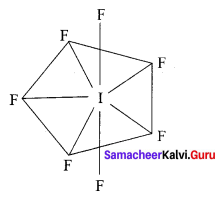Question 9.
Give the balanced equation for the reaction between chlorine with cold NaOH and hot NaOH.
Answer:
1. Reaction between chlorine with cold NaOH:
Cl2+ H2O → HCl + HOCl
HCl + NaOH → NaCl + H2O
HOCl + NaOH → NaOCl + H2O
Overall reaction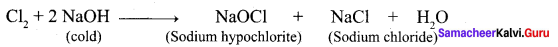Chlorine reacts with cold NaOH to give sodium chloride and sodium hypochlorite.

2. Reaction between chlorine with hot NaOH:
Cl2 + H2O → HCl + HOCl
HCl + NaOH → NaCl + H2O
HOCl + NaOH → NaOCl + H2O
3NaOCl → NaClO3+ 2NaCl
Overall reaction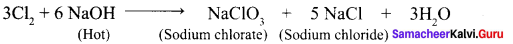Chlorine reacts with hot NaOH to give sodium chlorate and sodium chloride.

Question 10.
How will you prepare chlorine in the laboratory?
Answer:
1. Chlorine is prepared by the action of cone, sulphuric acid on chlorides in presence of manganese dioxide.
4NaCl + MnO2 + 4H2SO4 → Cl2 + MnCl2 + 4NaHSO4 + 2H2O

2. It can also be prepared by oxidising hydrochloric acid using various oxidising agents such as manganese dioxide, lead dioxide, potassium permanganate or dichromate.
PbO2 + 4HCl → PbCl2 + 2H2O + Cl2
MnO2 + 4HCl → MnCl2 + 2H2O + Cl2
2KMnO4 + 16HCl → 2KCl + 2MnCl + 8H2O + 5Cl2
K2Cr2O7 + 14HCl → 2KCl + 2CrCl3 + 7H2O + 3Cl2

3. When bleaching powder is treated with mineral acids chlorine is liberated
CaOCl2 + 2HCl → CaCl2 + H2O + Cl2
CaOCl2 + H2SO4 → CaSO4 + H2O + Cl2

Question 11.
Give the uses of sulphuric acid.
Answer:

1. Sulphuric acid is used in the manufacture of fertilisers, ammonium sulphate and super phosphates and other chemicals such as hydrochloric acid, nitric acid etc.
2. It is used as a drying agent and also used in the preparation of pigments, explosives etc.

Question 12.
Give a reason to support that sulphuric acid is a dehydrating agent.
Answer:
Sulphuric acid is highly soluble in water and has strong affinity towards water and hence it can be used as a dehydrating agent. When dissolved in water it forms mono ( H2SO4. H2O ) and di ( H2SO4. 2H2O ) hydrates and the reaction is exothermic. The dehydration property can also be illustrated by its reaction with organic compounds such as sugar, oxalic acid and formic acid.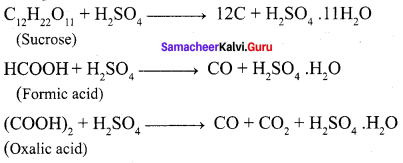Question 13.
Write the reason for the anamolous behaviour of Nitrogen.
Answer:
1. Due to its small size, high electro negativity, high ionisation enthalpy and absence of d-orbitals.

2. N, has a unique ability to form pπ – pπ multiple bond whereas the heavier members of this group (15) do not form pπ – pπ bond, because their atomic orbitals are so large and diffused that they cannot have effective overlapping.

3. Nitrogen exists a diatomic molecule with triple bond between the two atoms whereas other elements form single bond in the elemental state.

4. N cannot form dπ – pπ bond due to the absence of d – orbitals whereas other elements can.

Question 14.
Write the molecular formula and structural formula for the following molecules.
(a) Nitric acid
(b) dinitrogen pentoxide
(c) phosphoric acid
(d) phosphine
Answer: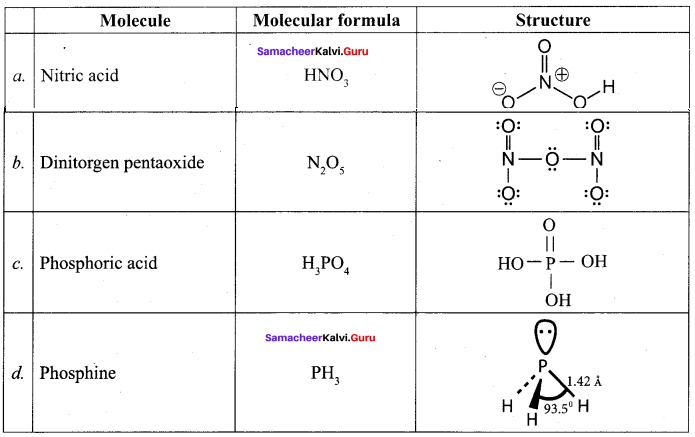Question 15.
Give the uses of argon.
Answer:
Argon prevents the oxidation of hot filament and prolongs the life in filament bulbs.

Question 16.
Write the valence shell electronic configuration of group-15 elements.
Answer:
General electronic configuration of group 15 elements are ns2np3.

1. Nitrogen – [He] 2s2 2p3
2. Phosphorous – [Ne] 3s2 3p3
3. Arsenic – [Ar] 3d10 4s2 4p3
4. Antimony – [Kr] 4d10 5s2 5p3
5. Bismuth – [Ne] 4f14 5s10 6s2 6p3

Question 17.
Give two equations to illustrate the chemical behaviour of phosphine.
Answer:
1. Phosphine reacts with halogens to give phosphorous penta halides.
PH3 + 4Cl2 → PCl5 + 3HCl

2. Phosphine forms coordination compound with lewis acids such as boron trichloride.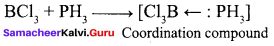3. Phosphine precipitates some metal from their salt solutions.
3AgNO3 + PH3 → Ag3P + 3HNO3

Question 18.
Give a reaction between nitric acid and a basic oxide.
Answer:
Nitric acid reacts with bases and basic oxides to form salts and water.

1. ZnO + 2HNO3 → Zn(NO3)2 + H2O
2. 3FeO + 10HNO3 → 3Fe(NO3)3 + NO + 5H2O

Question 19.
What happens when PCl5 is heated?
Answer:
On heating phosphorous pentachloride, it decomposes into phosphorus trichloride and chlorine.
PCl5 $$\underrightarrow { \triangle }$$ PCl3 + Cl2

Question 20.
Suggest a reason why HF is a weak acid, whereas binary acids of the all other halogens are strong acids.
Answer:
The hydrogen halides are extremely soluble in water due to the ionisation.
X + H2O → H3O+ + X
( X = F, Cl, Br or I )

Solutions of hydrogen halides are therefore acidic and known as hydrohalic acids. Hydrochloric, hydrobromic and hydroiodic acids are almost completely ionised and are therefore strong acids but HF is a weak acid. For HF,

• HF + H2O $$\rightleftharpoons$$ H3O+ + F
• HF + F → $${ HF }_{ 2 }^{ – }$$

At high concentration, the equilibrium involves the removal of fluoride ions is important. Since it affects the dissociation of hydrogen fluoride, therefore it is a weak acid.

Question 21.
Deduce the oxidation number of oxygen in hypofluorous acid – HOF.
Answer:
In case of O – F bond is HOF, fluorine is most electronegative element. So its oxidation number is -1. Thereby oxidation number of O is +1. Similarly in case of O – H bond is HOF. O is highly electronegative than H. So its oxidation number is -1 and oxidation number of H is +1. So, Net oxidation of oxygen is – 1 + 1 = 0.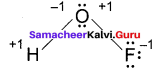Question 22.
What type of hybridisation occur in

1. BrF5
2. BrF3

Answer:
1. BrF5
BrF5 is a AX5 type. Therefore is has sp3d2 hybridisation. Hence, BrF5 molecule has square pyramidal shape.

2. BrF3
BrF3 is a AX3 type. Therefore it has sp3d hybridisation. Hence, BrF3 molecule has T-shape.

Question 23.
Complete the following reactions.
Answer: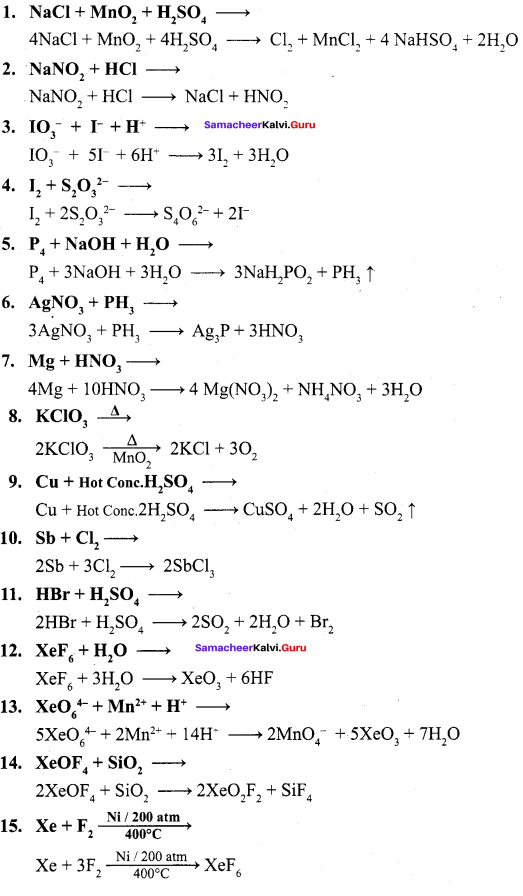Samacheer Kalvi 12th Chemistry p-Block Elements – II Evaluate yourself

Question 1.
Write the products formed in the reaction of nitric acid (both dilute and concentrated) with zinc.
Answer: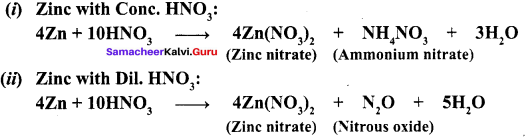### Samacheer Kalvi 12th Chemistry p-Block Elements – II Additional Questions

Samacheer Kalvi 12th Chemistry p-Block Elements – II 1 Mark Questions and Answers

I. Choose the best answer.

Question 1.
About 78% of earth atmosphere contains,…………
(a) P
(b) As
(c) N
(d) Bi
Answer:
(c) N

Question 2.
Which one of the following is not a pnictogens?
(a) Nitrogen
(b) Oxygen
(c) Phosphorous
(d) Antimony
Answer:
(b) Oxygen

Question 3.
Which one of the following shows isotopes?
(a) Nitrogen
(b) Arsenic
(c) Antimony
(d) Bismuth
Answer:
(a) Nitrogen

Question 4.
Nitrogen gas in atmosphere is separated industrially from liquid air by …………
(a) simple distillation
(b) Fractional distillation
(c) Sublimation
(d) Distillation under reduced pressure
Answer:
(b) Fractional distillation

Question 5.
Bond order for nitrogen molecule is …………
(a) 1
(b) 2
(c) 3
(d) 0
Answer:
(c) 3

Question 6.
Nitrogen gas is …………
(a) Inert
(b) Noble
(c) More reactive
(d) Less reactive
Answer:
(a) Inert

Question 7.
Which one of the following is used in cryosurgery?
(a) Liq N2
(b) Liq NH3
(c) Liq Na
(d) Liq H2
Answer:
(a) Liq N2

Question 8.
The dielectric constant of ammonia is (K) …………
(a) 10-30
(b) 10-14
(c) 1030
(d) 1014
Answer:
(a) 10-30

Question 9.
When ammonia reacts with copper sulphate solution to give complex, the colour of complex is …………
(a) violet
(b) deep blue
(c) blue
(d) Red
Answer:
(b) deep blue

Question 10.
H – N – H bond angle in NH3 is …………
(a) 109° 28’
(b) 107° 28’
(c) 104°
(d) 107°
Answer:
(d) 107°

Question 11.
Shape of ammonia is …………
(a) Planar
(b) Square planar
(c) Pyramidal
(d) Square pyramidal
Answer:
(c) Pyramidal

Question 12.
Nitric acid prepared in large scales using …………
(a) Ostwald’s process
(b) Haber’s process
(c) Contact process
(d) Deacon’s process
Answer:
(a) Ostwald’s process

Question 13.
Benzene undergoes nitration reaction to form nitrobenzene in this reaction takes place due to the formation of …………
(a) Hydronium ion
(b) Hydride ion
(c) Nitronium ion
(d) Nitrasonium ion
Answer:
(c) Nitronium ion

Question 14.
Oxidation state of N m FINO3 is…………
(a) ±2
(b) +3
(c) +4
(d) +5
Answer:
(d) +5

Question 15.
Compound used in photography is …………
(a) AgNO3
(b) AgBr
(c) AgCl
(d) AgI
Answer:
(a) AgNO3

Question 16.
Sodium nitrate
(a) Photography
(b) Firearms
(c) Royal water Sosurgerv …………
(d) Cryosurgery
Answer:
(b) Firearms

Question 17.
Nitrogen sesquoxide colour is …………
(a) colourless
(b) Brown
(c) Blue
(d) Red
Answer:
(c) Blue

Question 18.
White phosphorous is also called as …………
(a) Red phosphorous
(b) Black phosphorous
(c) Scarlet phosphorous
(d) Yellow phosphorous
Answer:
(d) Yellow phosphorous

Question 19.
White (Yellow) phosphorous glows in the dark due to oxidation which is called …………
(a) phosphorescence
(b) phosphorus
(c) Fluorescence
(d) Liminoscence
Answer:
(a) phosphorescence

Question 20.
Yellow phosphorous reacts with alkali on boiling in an inert atmosphere liberates …………
(a) Phosphorous acid
(b) Phosphoric acid
(c) Phosphine
(d) Pyrophosphoric acid
Answer:
(c) Phosphine

Question 21.
Consider the following statements.
(i) phosphine is the most important hydride of phosphorous
(ii) phosphine is a poisonous gas with rotten egg smell.
(iii) phosphine is a powerful reducing agent

Which of the above statement(s) is / are correct?
(a) (i) and (ii)
(b) (ii) and (iii)
(c) (i) and (iii)
(d) (ii) only
Ansswer:
(c) (i) and (iii)

Question 22.
When phosphine is heated with air it bums to gives …………
(a) Orthophosphoric acid
(b) Metaphosphoric acid
(c) Pyrophosphoric acid
(d) Phosphoroustrioxide
Answer:
(b) Metaphosphoric acid

Question 23.
Hybridisation of P in phosphine is …………
(a) sp3d
(b) sp3d2
(c) sp3d3
(d) sp3
Answer:
(d) sp3

Question 24.
Compounds used in Holme’s signal are …………
(a) Phosphine + Acetylene
(b) H3PO3+H3PO3
(c) Calcium carbide + calcium phosphide
(d) Calcium carbonate + calcium phosphate
Answer:
(c) Calcium carbide + calcium phosphide

Question 25.
Chalgogens are also called as …………
(a) Ore forming elements
(b) Group-16 elements
(c) group 17 elements
(d) Both (a) and (b)
Answer:
(d) Both (a) and (b)

Question 26.
Element present in the volcanic ashes is …………
(a) Oxygen
(b) Sulphur
(c) Selenium
(d) Tellurium
Answer:
(b) Sulphur

Question 27.
The decomposition of potassium chlorate speed up in the presence of …………
(a) MnO2
(h) Mn3O4
(c) MnSO4
(d) KMnO4
Answer:
(a) MnO2

Question 28.
Pure ozone is …………
(a) yellow gas
(b) blue gas
(c) Pale blue gas
(d) bright blue gas
Answer:
(c) Pale blue gas

Question 29.
Shape of ozone …………
(a) V-shape
(b) Linear shape
(c) bent shape
(d) spherical shape
Answer:
(c) bent shape

Question 30.
The rate of decompositon of ozone drops sharply in …………
(a) acidic medium
(b) alkaline medium
(c) neutral medium
(d) Ether medium
Answer:
(b) alkaline medium

Question 31.
Which one of the following used as fuel in rockets?
(a) Liq O2
(b) Liq CO2
(c) Liq N2
(d) Liq He – O2
Answer:
(a) Liq O2

Question 32.
Find out crystalline allotrophic form of sulphur?
(a) γ – sulphur
(b) λ – sulphur
(c) α – sulphur
(d) milk of sulphur
Answer:
(c) α – sulphur

Question 33.
Consider the following statements
(i) α – sulphur is the only thermodynamically stable allotrophic form.
(ii) At 140 ° C the mono clinic sulphur melts to form mobile pale yellow liquid called γ – sulphur
(iii) Monoclinic sulphur is stable between 96°C-119°C and slowly changes into λ- sulphur

Which of the above statement(s) is / are not correct?
(a) (i) only
(b) (ii) only
(c) (iii) only
(d) (ii) and (iii)
Answer:
(d) (ii) and (iii)

Question 34.
Sulphur di oxide, how many times heavier than air?
(a) 2 times
(b) 2.5 times
(c) 2.2 times
(d) 2.3 times
Answer:
(c) 2.2 times

Question 35.
Which one of following has temporary bleaching action?
(a) Chlorine
(b) SO3
(c) H3SO4
(d) SO2
Answer:
(d) SO2

Question 36.
Sulphuric acid can be manufactured by …………
(a) Ostwald’s process
(b) Lead chamber process
(c) Deacon’s process
(d) Haber’s process
Answer:
(b) Lead chamber process

Question 37.
Sulphuric acid is manufactured by contact process, catalyst used in contact process is …………
(a) V2O5
(b) TiCl4
(c) Fe
(d) Mo
Answer:
(c) V2O5

Question 38.
Benzene reacts with sulphuric acid to gives …………
(a) sulphate
(b) sulphide
(c) sulphonic acid
(d) sulphite
Answer:
(c) sulphonic acid

Question 39.
Reagent used to detect sulphate ion is …………
(a) BaCl2
(b) BaSO3
(c) (CH,COO),Pb
(d) both (a) and (c)
Answwer:
(d) both (a) and (c)

Question 40.
Deacon’s process is used to manufacture …………
(a) Cl2
(b) F2
(C) Br
(d) I2
Answer:
(a) Cl2

Question 41.
Catalyst used in Deacon’s process is …………
(a) CuCl2
(b) Cu2Cl2
(c) CuBr
(d) Cu2Br2
Answer:
(b) Cu2Cl2

Question 42.
C10H16 + 8C12 $$\underrightarrow { \triangle }$$ A. Identify A?
(a) Methane
(b) Ethane
(c) Carbon
(d) Propane
Answer:
(c) Carbon

Question 43.
Passing chlorine gas through dry slaked lime to produce …………
(a) CaOCl
(b) CaOCl2
(c) CaO
(d) CaCl2
Answer:
(b) CaOCl2

Question 44.
Which one of the following is used for purification of drinking water?
(a) SO3
(b) SO2
(c) Br2 / H2O
(d) Cl2
Answer:
(d) Cl2

Question 45.
Which one of the following is a weak acid?
(a) HF
(b) HCl
(c) HBr
(d) HI
Answer:
(a) HF

Question 46.
Reagent not stored in glass bottles?
(a) HCI
(b) HBr
(c) HF
(d) HI
Answer:
(c) HF

Question 47.
More reactive element is
(a) Fluorine
(b) Chlorine
(c) Bromine
(d) Iodine
Answer:
(a) Fluorine

Question 48.
The correct order of the acidity of hydrohalic acids?
(a) HF > HCI > HBr > HI
(b) HCI >HF >HBr >HI
(c) HBr > HCI >HF > HI
(d) HI > HBr > HCI > HF
Answer:
(d) HI > HBr > HCI > HF

Question 49.
Consider the following statements
(i) In interhalogen compounds, the central atom will be the smaller one.
(ii) It can be formed only between two halogen and not more than two halogens.
(iii) They are strong reducing agents.

Which of the above statement(s) is / are not correct?
(a) (i) only
(b) (ii) and (iii)
(c) (i) and (iii)
(d) (iii) only
Answer:
(c) (i) and (iii)

Question 50.
Shape of ClF3 is …………
(a) Linear
(b) T-shape
(c) Pyrimidal
(d) Square planar
Answer:
(b) T-shape

Question 51.
Which one of the following is more acidic?
(a) HOCl
(b) HCIO2
(c) HClO3
(d) HClO4
Answer:
(d) HClO4

Question 52.
When XeF6 reacts with 2.5 M NaOH gives …………
(a) Na4XeO6
(b) Na2XeO3
(c) XeO2F2
(d) XeO3
Answer:
(a) Na4XeO6

Question 53.
Shape of XeF6 is …………
(a) Octahedron
(b) Distorted octahedron
(c) Pyramidal
(d) Tetrahedron
Answer:
(b) Distorted octahedron

Question 54.
Which one of the following can penetrate through dense fog?
(a) He
(b) Ne
(c) Kr
(d) Rn
Answer:
(c) Kr

Question 55.
Find out radioactive element?
(a) He
(b) Rn
(c) Xe
(d) Ar
Answer:
(b) Rn

II. Fill in the blanks:

1. The 11th most abundant element is ………….
2. …………. is the principle gas of atmosphere.
3. Nitrogen is chemically ………….
4. …………. process for the synthesis of ammonia.
5. …………. is used for the manufacture of calcium cyanamide
6. …………. is a pungent smelling gas.
7. Ammonia acts as a …………. agent.
8. With excess of chlorine, ammonia reacts to give …………. an explosive substance.
9. When excess ammonia is added to aqueous solution of copper sulphate …………. colour compound is formed.
10. Pure nitric acid becomes …………. on standing.
11. …………. is used in gunpower for firearms.
12. The decomposition of ammonium nitrate gives ………….
13. White phosphorous is colourless but becomes pale yellow due to formation of a …………. upon standing.
14. The white phosphorous can be changed into …………. by heated it to 420°C in the absence of air and light.
15. …………. reacts with alkali on boiling in an inert atmosphere liberating phosphine.
16. …………. is used in the match boxes.
17. Phosphine is …………. smelling gas.
18. Phosphine has a …………. shape.
19. …………. is used for producing smoke screen.
20. When phosphorous trichloridie is hydrolysed with cold water it gives ………….
21. Elements belonging group 16 are called ………….
22. Under ordinary condition oxygen exists as a …………. gas.
23. Allotrophic form of oxygen is …………. and ………….
24. Pure ozone is …………. gas.
25. …………. is used in welding purpose.
26. Monoclinic sulphur is stable between 96° and 119°C and slowly changes into ………….
27. …………. gas is found in volcanic eruptions.
28. A large amount of …………. gas is released into atmosphere from plants using coal and oil and copper melting plants.
29. Sulphurdioxide gas has …………. odour.
30. Sulphurdioxide can be used for …………. and …………. in agriculture.
31. In SO3 S-atom undergoes …………. hybridisation.
32. In SO3 a double bond arises between S and O is due to …………. overlapping.
33. High boiling point and viscosity of sulphuric acid is due to ………….
34. …………. is used as a drying agent.
35. The main source of fluorine is ………….
36. The main source of chlorine is ………….
37. Chlorine is a …………. gas.
38. Chlorine is soluble in water and its solution is referred as ………….
39. …………. is produced by passing chlorine gas through dry slaked lime.
40. …………. is used in extraction of gold and platinum.
41. …………. is used for extraction of glue from bone.
42. At room temperature, hydrogen halides are gases but …………. can be readily liquefied.
43. Liberation of iodine which gives a …………. colouration with starch.
44. Each halogen combines with other halogens to form a series of compounds called ………….
45. Structure of AX7 type is ………….
46. Oxidation state of Cl in HClO4 is ………….
47. Noble gases have the …………. ionisation energy.
48. Xenon reacts with PtF6 and gave an ………….
49. Kr and fluorine gases are irradiated with SbF it forms ………….
50. Shape of XeOF4 is ………….
51. Helium used for filling air ………….
52. …………. is used in fluorescent bulbs.
53. …………. is used in high speed electronic flash bulbs.
54. Radon is a source of …………. rays.
55. …………. is formed by the hydrolysis of urea.
56. …………. element subtimes at 889 K.
57. Yellow phosphorus has a characteristics …………. smell.

Answers:

1. phosporous
2. Nitrogen
3. Inert
4. Haber’s
5. Nitrogen
6. Ammonia
7. Reducing
8. Nitrogen trichloride
9. Deep blue
10. Yellow
11. Nitric acid / NaNO
12. Nitrous oxide
13. Layer of red phosphorous
14. Red phosphorous
15. White phosphorous
16. Red phosporous
17. Rotten fish
18. Pyramidal
19. Phosphine
20. phosporous acid
21. Chalgogens
22. diatomic
23. dioxygen and ozone
24. pale blue
25. Oxyacetylene
26. Rhombic sulphur
27. Sulphurdioxide
28. SO2
29. Suffocating
30. disinfecting crops and plants
31. sp2
32. pπ – dπ
33. Hydrogen bonding
34. Sulphuric acid
35. Fluorite
36. Sodium chloride
37. Green yellow
38. Chlorine water
39. Bleaching powder
40. Chlorine
41. Hydrochloric acid
42. hydrogen fluroide
43. blue-black
44. Inter-halogen compounds
45. pentagonal bipyramidal
46. +7
47. largest
48. orange yellow solid [XePtF6]
49. KrF2. 2SbF3
50. Square pyramidal
51. Balloons
52. Krypton
53. Xenon
54. Gamma
55. Ammonia
56. Arsenic
57. Garlic

II. Match the following:

Question 1.
(i) Haber’s process – (a) HNO3
(ii) Deacon’s process – (b) Ammonia
(iii) Contact process – (c) Chlorine
(iv) Ostwald’s process – (d) H2SO4
Answer:
(i) b
(ii) c
(iii) d
(iv) a

Question 2.
(i) Nitric acid – (a) Purification of bone black
(ii) HCl – (b) Photography
(iii) White (yellow) phosphorous – (c) Rotten fish smell
(iv) Phosphine – (d) Phosphorescence
Answer:
(i) b
(ii) a
(iii) d
(iv) c

Question 3.
(i) Nitrogen sesquoxide – (a) H2N2O2
(ii) Nitrous oxide – (b) H4N2O4
(iii) Hyponitrous acid – (c) N2O
(iv) Hydronitrous acid – (d) N2O3
Answer:
(i) d
(ii) c
(iii) a
(iv) b

Question 4.
(i) N2O – (a) +5
(ii) N2O4 – (b) +3
(iii) N2O5 – (c) +1
(iv) N2O3 – (d) +4
Answer:
(i) c
(ii) d
(iii) a
(iv) b

Question 5.
(i) White phosphorous – (a) Volcanic eruptions
(ii) Red phosphorous – (b) Yellow phosphorous
(iii) Phosphine – (c) Match boxes
(iv) SO2 – (d) smoke screen
Answer:
(i) b
(ii) c
(iii) d
(iv) a

Question 6.
(i) ammonia – (a) suffocating odour
(ii) SO2 – (b) Rotten fish smell
(iii) PH3 – (c) Greenish yellow gas
(iv) Cl2 – (d) pungent smelling gas
Answer:
(i) d
(ii) a
(iii) b
(iv) c

Question 7.
(i) XeF4 – (a) sp3
(ii) XeOF2 – (b) sp3d2
(iii) XeO3 – (c) sp3d3
(iv) XeF – (d) sp3d
Answer:
(i) b
(ii) d
(iii) a
(iv) c

Question 8.
(i) Helium – (a) flash bulbs
(ii) Neon – (b) radioactive
(iii) Krypion – (c) air balloons
(iv) Radon – (d) Brilliant red
Answer:
(i) c
(ii) d
(iii) a
(iv) b

IV. Assertion and Reason

Question 1.
Assertion (A) – Xenon is used in high speed electronic flash bulbs used by photographers.
Reason (R) – Xenon emits an intense light in discharge tubes instantly.
(a) A and R are correct and R explains A
(b) A and R are correct but doesn’t explains A
(c) A is correct but R is wrong
(d) A is wrong but R is correct
Answer:
(a) A and R are correct and R explains A

Question 2.
Assertion (A) – Noble gases have the largest ionisation energy compared to any other elements.
Reason (R) – Noble gases have incomplete filled orbital.
(a) A and R are correct and R explains A
(b) A and R are correct but doesn’t explains A
(c) A is correct but R is wrong
(d) A is wrong but R is correct
Answer:
(c) A is correct but R is wrong

Question 3.
Assertion (A) – Hydrogen iodide decomposes at 400<sup>0</sup>C while hydrogen fluoride and hydrogen chloride are stable at this temperature.
Reason (R) – Thermal stability of hydrogen halides decreases from fluoride to iodide.
(a) A and R are correct and R explains A
(b) A and R are correct but doesn’t explains A
(c) A is correct but R is wrong
(d) A is wrong but R is correct
Answer:
(a) A and R are correct and R explains A

Question 4.
Assertion (A) – The bleaching of chlorine is temporary.
Reason (R) – Chlorine oxidises ferrous salts to ferric salts.
(a) A and R are correct and R explains A
(b) A and R are correct but doesn’t explains A
(c) A is correct but R is wrong
(d) A is wrong but R is correct
Answer:
(d) A is wrong but R is correct

Question 5.
Assertion (A) – Sulphuric acid is highly reactive.
Reason (R) – Sulphuric acid can act as strong acid and an oxidising agent.
(a) A and R are correct and R explains A
(b) A and R are correct but doesn’t explains A
(c) A is correct but R is wrong
(d) A is wrong but R is correct
Answer:
(a) A and R are correct but doesn’t explains A

Question 6.
Assertion (A) – Sulphuric acid is a high boiling point and viscous liquid.
Reason (R) – This is due to the association of molecules together through hydrogen bonding.
(a) A and R are correct and R explains A
(b) A and R are correct but doesn’t explains A
(c) A is correct but R is wrong
(d) A is wrong but R is correct
Answer:
(a) A and R are correct and R explains A

Question 7.
Assertion (A) – Monoclinic sulphur is less stable than rhomobic sulphur.
Reason (R) – Monoclinic sulphur is stable between 96°C – 119°C and slowly changes into rhombic sulphur.
(a) A and R are correct and R explains A
(b) A and R are correct but doesn’t explains A
(c) A is correct but R is wrong
(d) A is wrong but R is correct
Answer:
(a) A and R are correct and R explains A

Question 8.
Assertion (A) – Nitrogen gas is chemically inert.
Reason (R) – Nitrogen has low bonding energy.
(a) A and R are correct and R explains A
(b) A and R are correct but doesn’t explains A
(c) A is correct but R is wrong
(d) A is wrong but R is correct
Answer:
(c) A is correct but R is wrong

V. Find the odd one out
Question 1.
(a) NO
(b) HNO3
(C) NO2
(d) N2O
Answer:
(b) HNO3
Hint: HNO3 is acid and other are oxides.

Question 2.
(a) Nitrous acid
(b) Nitric acid
(c) Hyponitrous acid
(d) Pemitrous acid
Answer:
(d) Pemitrous acid
Hint: Pemitrous acid contains peroxide linkage others doesn’t have peroxide linkage.

Question 3.
(a) White phosphorous
(b) Red phosphorous
(c) phosphorous pentaoxide
(d) black phosphorous
Answer:
(c) phosphorous pentaoxide
Hint: P2O5 is a compound of phosphorous and others are allotropic form of phosphorous.

Question 4.
(a) PH3
(b) HPO3
(c) H3PO3
(d) H3PO4
Answer:
(a) PH3
Hint: PH3 is hydrides of phosphorous and others are oxo acids of phosphorous.

Question 5.
(a) He
(b) Ne
(c) Ar
(d) Xe
Answer:
(d) Xe
Hint: Xe forms several chemical compounds than others.

VI. Find out the correct pair.

Question 1.
(a) Helium – filament bulbs
(b) Krypton – prevent bonds
(c) Xenon – Lasers
(d) Radon – flash bulbs
Answer:
(c) Xenon – Lasers

Question 2.
(a) Ra – gamma rays
(b) Xe – cancer growth
(c) Ne – balloons
(d) Kr – advertisement bulb
Answer:
(a) Ra – gamma rays

Question 3.
(a) ClF3 – Linear
(b) BrF5 – T Shaped
(c) IF4 – square pyrimidal
(d) BrF5 – square pyrimidal
Answer:
(d) BrF5 – square pyrimidal

Question 4.
(a) XeOF2 – sp3
(b) XeF6 – sp3d3
(c) XeF4 – sp3
(d) XeOF4 – sp3d
Answer:
(b) XeF6 – sp3d3

Question 5.
(a) OF2 = -1
(b) Cl4O4 = -1
(c) I2O4 = -1
(d) I2O9 = -1
Answer:
(a) OF2 = -1

Question 6.
(a) Royal water – Dissolving gold
(b) Chlorine – Entraction of glue from bone
(c) HCl- Extraction of gold
(d) Chlorine – Temporary bleaching
Answer:
(a) Royal water – Dissolving gold

Question 7.
(a) O – 2s22p3
(b) S – 3s23p4
(c) Se – 4d105s25p4
(d) Te – 3d104s24p4
Answer:
(b) S – 3s23p4

VI.Find out the incorrect pair.

Question 1.
(a) Nitrogen gas – inert
(b) Ammonia – pungent smelling gas
(c) Nitric acid – oxidizing agent
(d) Phosphine – rotten egg smell
Answer:
(d) Phosphine – rotten egg smell

Question 2.
(a) Liquid nitrogen – biological preservation
(b) Nitric acid – photography
(c) white phosphorous – yellow phosphorous
(d) phosphorous – welding
Answer:
(d) phosphorous – welding

Question 3.
(a) N2O = +1
(b) N2O = +2
(c) N2O3 = +5
(d) NO2 = +4
Answer:
(c) N2O3 = +5

Question 4.
(a) Hvponitrous acid – N2O
(b) Nitrous acid – HNO2
(c) pernitric acid – HNO4
(d) pernitrous acid – HOONO
Answer:
(a) Hvponitrous acid – N2O

Question 5.
(a) PH3 – Holme’s signal
(b) O2 – welding
(c) H2SO4 – Disinfecting crops
(d) SO3 – Bleaching hair
Answer:
(c) H2SO4 – Disinfecting crops

Question 6.
(a) H2SO4 drying agent
(b) Chlorine – Deacon’s process
(c) HCI – purification of bone black
(d) Helium – flash bulbs
Answer:
(d) Helium – flash bulbs

Question 7.
(a) ICl – Linear
(b) CIF3 – T shape
(c) IF5 – pentagonal bipyramidal
(d) IF7 – pentagonal bipyramidal
Answer:
(c) IF5 – pentagonal bipyramidal

Question 8.
(a) HOCl = +2
(b) HOCl = +3
(e) HOCI = +5
(d) HOCl4 = +7
Answer:
(a) HOCl = +2

Question 9.
(a) XeF4 – sp3d3
(b) XeF6 – sp3d3
(e) XeOF4 – sp3d2
(d) XeO3 – sp3d
Answer:
(d) XeO3 – sp3d

Question 10.
(a) He – cryogenics
(b) Ne – advertisement
(c) Kr – flurescent bulbs
(d) Ra – Lasers.
Answer:
(d) Ra – Lasers.

Samacheer Kalvi 12th Chemistry p-Block Elements – II 2 Mark Questions and Answers

Question  1.
What are pnictogens?
Answer:
The group – 15 elements like nitrogen, phosphorous Arsenic, Antimony and Bismuth are collectively called as pnictogens. Their general outer electronic configuration is ns2np3.

Question 2.
What happen when sodium azide undergoes thermal decomposition?
Answer:
Pure nitrogen gas can be obtained by the thermal decomposition of sodium azide about 575K
2NaN3 $$\underrightarrow { 575K }$$ 2Na + 3N2

Question 3.
How will you prepare ammonia from nitrogen? and mention the name of process?
Answer:
Nitrogen directly reacts with hydrogen gives ammonia. This reaction is favoured by high pressures and at optimum temperature in the presence of iron catalyst,
N2 + 3H3 $$\rightleftharpoons$$ 2NH3
This process is called as Haber’s process.

Question 4.
Why nitrogen gas is chemically inert?
Answer:
The chemically inert character of nitrogen is largely due to high bonding energy of the molecules 225 cal mol-1 .Interestingly the triply bonded species is notable for its less reactivity in comparison with other iso-electronic triply bonded systems such as -C $$\equiv$$ C – C $$\equiv$$ Q, X- C $$\equiv$$ N, etc.

Question 5.
Mention the uses of nitrogen?
Answer:

1. Nitrogen is used for the manufacture of ammonia, nitric acid and calcium cyanamide etc.
2. Liquid nitrogen is used for producing low temperature required in cryosurgery, and so in biological preservation

Question 6.
Explain the action of heat on ammonia.
Answer:
Above 500°C ammonia decomposes into its elements. The decomposition may be accelerated by metallic catalysts like Nickel, Iron. Almost complete dissociation occurs on continuous sparking.
2NH3 $$\underrightarrow { { 500 }^{ 0 }C }$$ N2 + 3H2

Question 7.
Prove ammonia act as a reducing agent?
Answer:
Ammonia reduces the metal oxides to metal when passed over heated metallic oxide.
3PbO + 2NH3 → 3Pb + N2 + 3H2O

Question 8.
What happen when copper sulphate reacts with ammonia?
Answer:
When excess ammonia is added to aqueous solution copper sulphate a deep blue colour Complex [Cu(NH3)4 ]2+ is formed.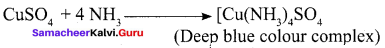Question 9.
Pure nitric acid is colourless, on standing it becomes yellow. Justify your answer.
Answer:
Nitric acid decomposes on exposure to sunlight or on being heated, into nitrogen dioxide, water and oxygen.
4HNO4 → 4NO2 + 2H2O + O2
Due to this reaction pure acid or its concentrated solution becomes yellow on standing.

Question 10.
Write the products formed in the reaction of nitric acid with dilute and concentrated with magnesium.
Answer:
1. Magnesium with cone. HNO3:
4Mg + 10HNO3 → 4Mg(NO3)2 + NH4NO3 + 3H2O
Magnesium reacts with concentraated nitric acid to gives both magnesium nitrate and ammonium nitrate.

2. Magnesium with dil HNO3:
Magnesium reacts with dilute nitric acid to gives both magnesium nitrate and nitrous oxide.
4Mg + 10HNO3 → 4 Mg(NO3)2 + N2O + 5H2O

Question 11.
Give the uses of nitric acid.
Answer:

1. Nitric acid is used as a oxidising agent and in the preparation of aquaregia.
2. 4. Salts of nitric acid are used in photography (AgNO3) and gunpowder for firearms. (NaNO3) .

Question 12.
How will you prepare nitric oxide from sodium nitrite?
Answer:
When sodium nitrite reacts with ferrous sulphate in the presence of sulphuric acid to gives nitric oxide.
2NaNO2 + 2FeSO4 + 3H2S → Fe2(SO4)3 + 2NaHSO4 + 2H2O + 2NO

Question 13.
How will you prepare nitrogen pentoxide?
Answer:
Nitric acid reacts with phosphorous pentaoxide to give nitrogen pentoxide.
2HNO3 + P2O5 → N2O5 + 2HPO3

Question 14.
Draw the structure of

1. N2O
2. N2O3

Answer:
1. N2O (Nitrous oxide)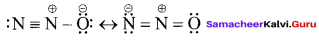2. N2O3 (Nitrogen sesquoxide)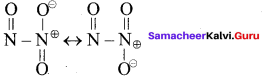Question 15.
Draw- the structure of

1. N2O4
2. N2O3

Answer:
1. N2O4 (Nitrogen tetraoxide)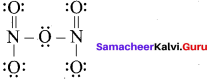2. N2O5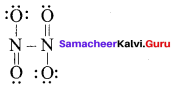Question 16.
Draw the structure of

1. Hyponitrous acid
2. Hydronitrous acid.

Answer:
(a) Hypon trous acid – H2N2O2
HO – N = H – OH

(b) Hydronitrous acid: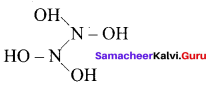Question 17.
Mention the allotropic forms of phosphorous?
Answer:
The most common allotropic forms of phosphorous

1. white phosphorous
2. Red phosphorous
3. Black phosphorous

Question 18.
Why white phosphorous is also known as yellow phosphorous?
Answer:
The freshly prepared white phosphorus is colourless but becomes pale yellow due to formation of a layer of red phosphorus upon standing. Hence it is also known as yellow phosphorus.

Question 19.
What is phosphorescence?
Answer:
White (yellow) phosphorous glows in the dark due to oxidation which is called phosphorescence.

Question 20.
Why white phosphorous undergoes spontaneous combustion in air?
Answer:
White phosphorous ignition temperature is very low and hence it undergoes spontaneous combustion in air at room temperature and during in the combusition process, white phosphorous produces P2O5.

Question 21.
Draw the structure of

1. white phosphorous
2. red phosphorous

Answer:
1. White phosphorous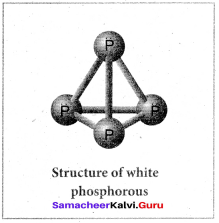2. Red phosphorous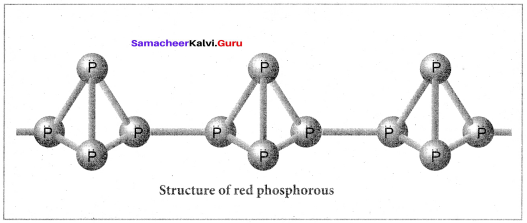Question 22.
How will you convert red phosphorous into P2O3 and P2Q5?
Answer:
Red phosphorus reacts with oxygen on heating to give phosphorus trioxide or phosphorus pentoxide.
P4 + 3O2 $$\underrightarrow { \triangle }$$ P4O6(phosphorous trioxide)
P4 + 5O2 $$\underrightarrow { \triangle }$$ P4Q10 (phosphorous pentaoxide)

Question 23.
How will you prepare orthophosphoric acid from phosphorous?
Answer:
When phosphorous is treated with cone.nitric acid it is oxidised to orthophosphoric acid. This reaction is catalysed by iodine crystals.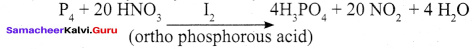Question 24.
Mention the uses of phosphorous?
Answer:

1. The red phosphorus is used in the match boxes.
2. It is also used for the production of certain alloys such as phosphor bronze.

Question 25.
Show that phosphine is weakly basic?
Answer:
Phosphine is weakly basic and forms phosphonium salts with halogen acids.
PH3 +HI → PH4I
PH4I + H2O $$\underrightarrow { \triangle }$$ PH3+ H3O + I

Question 26.
Draw the structure of PCl3
Answer: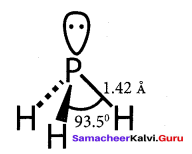Question 27.
How will you prepare PCl3 from white phosphorous?

1. When a slow stream of chlorine is passed over white phosphorous, PCl3 is formed
2. It can also be obtained by treating white phosphorous with thionyl chloride.

Question 28.
What happen when PCl3 is treated with cold water?
Answer:
When PCl3 is hydrolysed with cold water it gives phosphorous acid.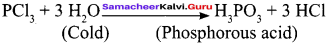Question 29.
How will you prepare PCl3 ?
Answer:
When PCl3 is treated with excess chlorine, phosphorous pentachloride is obtained.
PCl3+ Cl2 → PCl5

Question 30.
Mention the uses of PCl3 and PCl5?
answer:

1. Phosphorus trichloride is used as a chlorinating agent and for the preparation of H2PO3.
2. Phosphorous pentachloride is a chlorinating agent and is useful for replacing hydroxyl groups by chlorine atom.

Question 31.
Draw the structure of H3PO2 and H3PO3?
Answer: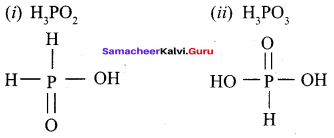Question 32.
Draw the structure of H4P2O6 and H4P2O3?
Answwer:
(i) H4P2O6
(ii) H4P2O3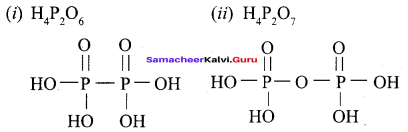Question 33.
Give the method to prepare hypophosphorous acid and pyrophosphoric acid?
Answer:
1. Hypophosphorous acid – Phosphorous reacts with water to give hypophosphorous acid.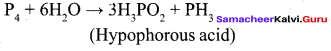2. Pyrophosphoric acid – Phosphorous acid is heated they produce pyrophosphoric acid.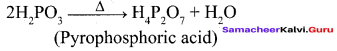Question 34.
Complete the reactions

1. HgO $$\underrightarrow { \triangle }$$ ?
2. BaO2 $$\underrightarrow { \triangle }$$ ?

Answer:

1. HgO $$\underrightarrow { \triangle }$$ 2Hg + O2
2. 2BaO2 $$\underrightarrow { \triangle }$$ 2BaO + O2

Question 35.
Give and explain the reaction used to estimation of ozone.
Answer:
Ozone oxidises potassium iodide to iodine.
O3 + 2KI + H2O → 2KOH + O2 + I2
This reaction is quantitative and can be used for estimation of ozone.

Question 36.
Write a short notes on Rhombic sulphur?
Answer:
Rhombic sulphur also known as a sulphur, is the only thermodynamically stable allotropic form at ordinary temperature and pressure. The crystals have a characteristic yellow colour and composed of S8 molecules. When heated slowly above 96°C, it converts into monoclinic sulphur. Upon cooling below 96°C the β form converts back to a form.

Question 37.
Write a notes on monoclinic sulphur?
Answer:
Monoclinic sulphur also contains S8 molecules in addition to small amount of S6 molecules. It exists as a long needle like prism and is also called as prismatic sulphur. It is stable between 96° – 119°C and slowly changes into rhombic sulphur.

Question 38.
What are λ – sulphur?
Answer:
Sulphur also exists in liquid and gaseous states. At around 140° C the monoclinic sulphur melts to form mobile pale yellow liquid called λ sulphur.

Question 39.
How will you prepare sulphurdioxide by laboratory method?
Answer:
Sulphur dioxide is prepared in the laboratory treating a metal or metal sulphite with sulphuric acid.
Cu + 2H2SO4 → CuSO4 + SO2 + 2H2O
$${ SO }_{ 3 }^{ – }$$ + 2H+ → H2O + SO2

Question 40.
Complete the reactions,
Answer:

1. Zns + O2 $$\underrightarrow { \triangle }$$ ?
2. FeS2 + O2 $$\underrightarrow { \triangle }$$ ?

Answer:

1. 2Zns + 3O2 $$\underrightarrow { \triangle }$$ 2ZnO + 2SO2
2. 4FeS2 + 11O2 $$\underrightarrow { \triangle }$$ 2Fe2O3 + 8SO2

Question 41.
What happen when sulphurdioxdie reacts with sodium hydroxide and sodium carbonate?
Answer:
Sulphur dioxide reacts with sodium hydroxide and sodium carbonate to form sodium bisulphite and sodium sulphite respectively.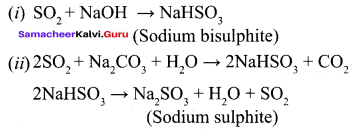Question 42.
Mention the uses of sulphurdioxide?
Answer:

1. Sulphur dioxide is used in bleaching hair, silk, wool etc…
2. It can be used for disinfecting crops and plants in agriculture.

Question 43.
Why sulphuric acid is high boiling and viscous liquid ?
Answer:
Sulphuric acid is high boiling and viscous liquid this is due to the association of molecules together through hydrogen bonding.

Question 44.
Explain the reaction between benzene and sulphuric acid.
Answer:
Sulphuric acid reacts with benzene to give benzene sulphuric acid.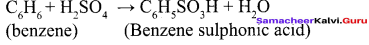Question 45.
Give the uses of sulphuric acid?
Answer:

1. Sulphuric acid is used in the manufacture of fertilisers, ammonium sulphate and super phosphates and other chemicals such as hydrochloric acid, nitric acid etc…
2. It is used as a drying agent and also used in the preparation of pigments, explosives etc..

Question 46.
Draw the structure of H2SO3 and H2SO4 ?
Answer:
1. H2SO3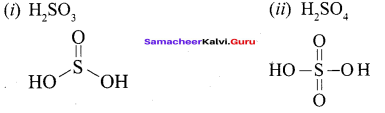2. H2SO4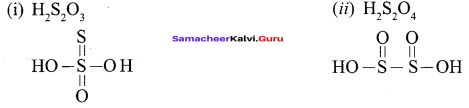Question 48.
Draw the structure of

1. Pyrosulphuric acid
2. Peroromonosulphuric acid?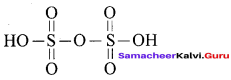Samacheer Kalvi 12th Chemistry Solutions Chapter 3 p-Block Elements – II

Answer:
1. Pyrosulphuric acid H2S2O7

2. Peroromonosulphuric acid H2SO5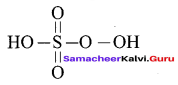Question 49.
What happen when chlorine reacts with trupentine?
Answer:
When chlorine burnt with turpentine it forms carbon and hydrochloric acid.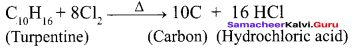Question 50.
How will you prepare hydrochloric acid by laboratory method?
Answer:
It is prepared by the action of sodium chloride and concentrated sulphuric acid

• NaCl + H2SO4 → NaHSO4 + HCl
• NaHSO4 + NaCl → Na2SO4 + HCl

Dry hydrochloric acid is obtained by passing the gas through conc. sulphuric acid

Question 51.
Mention the uses of hydrochloric acid?
Answer:

1. Hydrochloric acid is used for the manufacture of chlorine, ammonium chloride, glucose from com starch etc.,
2. It is used in the extraction of glue from bone and also for purification of bone black.

Question 52.
Complet the following reaction?
Answer: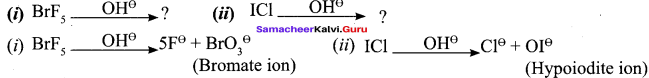Question 53.
Why noble gases have the largest ionisation energy?
Answer:
Noble gases have the largest ionisation energy compared to any other elements in a given row as they have completely filled orbital (ns2np6) in their outer most shell. They are extremely stable and have a small tendency to gain or lose electrons. Hence noble gases have the largest ionisation energy.

Question 54.
Mention the uses of Neon?
Answer:
Neon is used in advertisement as neon sign and the brilliant red glow is caused by passing electric current through neon gas under low
pressure.

Question 55.
Give the uses of Krypton?
Answer:

1. Krypton is used in fluorescent bulbs, flash bulbs etc…
2. Lamps filed with krypton are used in airports as approaching lights as they can penetrate through dense fog.

Question 56.
Mention the application of Xenon?
Answer:

1. Xenon is used in fluorescent bulbs, flash bulbs and lasers.
2. Xenon emits an intense light in discharge tubes instantly. Due to this it is used in high speed electronic flash bulbs used by photographers.

Question 57.
Give the uses of Radon?
Answer:

1. Radon is radioactive and used as a source of gamma rays
2. Radon gas is sealed as small capsules and implanted in the body to destroy malignant i.e. cancer growth.

Question 58.
Why are pentahalides more covalent than trihalides?
Answer:
Since elements in the +5 oxidation state have less tendency to lose electrons than in the +3 oxidation state, therefore elements in the +5 oxidation state are more covalent than in the +3 oxidation state. In other words, pentahalides are more covalent than trihalides.

59. Why is N2 less reactive at room temperature?
Answer:
Due to the presence of triple bond between the two nitrogen atoms, the bond dissociation energy of N2 (941.4 kJ mol) is very high. Therefore, N2 is less reactive at room temperature.

Question 60.
Why is ICl more reactive than I2 ?
Answer:
ICl bond is weaker than I – I bond. Therefore ICl can break easily to form halogen atoms which readily brings about the reaction.

Question 61.
Why is helium used in diving apparatus?
Answer:
It is used as a diluent for oxygen in modern diving apparatus because of its very low solubility in blood.

Question 62.
Give the disproportionation reaction of H3PO3?
Answer:
H3PO3 on heating undergoes self-oxidation reduction as: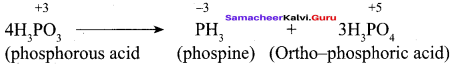Question 63.
Why is red Phosphorus less reactive than white Phosphorus?
Answer:
White Phosphorus is more reactive than red Phosphorus under normal conditions because of angular strain in the P4 molecule where the angles are only 60°.

Question 64.
Nitrogen does not form any pentahalide like Phosphorus. Why?
Answer:
Nitrogen does not form pentahalide due to non-availability of the d-orbitals in its valence shell.

Question 65.
Give reason for the following. Among the noble gases only Xenon is well known to form chemical compounds?
Answer:
Xe is largest in size and has the highest polarising power.

Question 66.
Why is hydrogen sulphide, with greater molar mass a gas, while water a liquid at room temperature?
Answer:
H2O molecules are associated with intermolecular H-bonding, H2S is not because oxygen is more electronegative and smaller in size than sulphur. That is why H2O is a liquid and H2S is a gas.

Question 67.
Noble gases are chemically inert. Give reasons.
Answer:
Noble gases are chemically inert because they have their octet complete except Helium, i.e. they have a stable electronic configurations.

Question 68.
Why do noble gases exist as monoatomic?
Answer:
Noble gases have stable electronic configuration, that is why they have no tendency to lose or gain electrons.Therefore they do not form covalent bond.

Question 69.
Nitrogen exists as diatomic molecule and Phosphrus as P4. Why?
Answer:
Nitrogen has a triple bond between its two atoms because of its small size and high electro negativity. Phosphorus P4 has single bond, that is why it is tetra-atomic.

Question 70.
Why is H2S more acidic than water?
Answer:
It is because bond dissociation energy of S – H bond is less than O – H bond due to longer bond length.

Samacheer Kalvi 12th Chemistry p-Block Elements – II 3 Marks Questions and Answers

Question 1.
Complete the following reactions.
(i) 6Li + N2 → ?
(ii) 3Ca + N2 → ?
(iii) 2B + N2 → ?
Answer: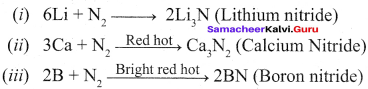Qustion 2.
How is ammonia prepared?
Answer:
1. Ammonia is formed by the hydrolysis of urea.
NH2CONH2 + H2O → 2NH3 + CO2

2. Ammonia is prepared in the laboratory by heating an ammonium salt with a base.
$${ 2NH }_{ 4 }^{ + }$$ + OH → 2NH3+ H2O
2NH4Cl + CaO → CaCl2 + 2NH3 + H2O

3. It can also be prepared by heating a metal nitrides such as magnesium nitride with water.
Mg3N2+ 6H2O → 3Mg(OH)2 + 2NH3

Question 3.
Explain the structure of ammonia.
Answer:
Ammonia molecule is pyramidal in shape N – H bond distance is 1.016 A and H – H bond distance is 1.645 A with a bond angle 107°. The structure of ammonia may be regarded as a tetrahedral with one lone pair of electrons in one tetrahedral position hence it has a pyramidal shape as shown in the figure.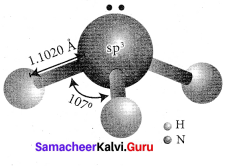Question 4.
How will you prepare nitric acid?
Answer:
Nitric acid is prepared by heating equal amounts of potassium or sodium nitrate with concentrated sulphuric acid.
KNO3 + H2SO4 → KHSO4 + HNO3

The temperature is kept as low as possible to avoid decomposition of nitric acid. The acid condenses to a fuming liquids which is coloured brown by the presence of a little nitrogen dioxide which is formed due to the decomposition of nitric acid.
4HNO3 → 4NO2 + 2H2O + O2

Question 5.
Discuss the Commercial method to prepare Nitric acid.
(Or)
How will you prepare nitric acid by Ostwald’s process?
Answer:
Nitric acid prepared in large scales using Ostwald’s process. In this method ammonia from Haber’s process is mixed about 10 times of air. This mixture is preheated and passed into the catalyst chamber where they come in contact with platinum gauze. The temperature rises to about 1275 K and the metallic gauze brings about the rapid catalytic oxidation of ammonia resulting in the formation of NO, which then oxidised to nitrogen dioxide.
4NH3 + 5O2 → 4NO + 6H3O + 120kJ
2NO + O2 → 2NO3

The nitrogen dioxide produced is passed through a series of adsorption towers. It reacts with water to give nitric acid. Nitric acid formed is bleached by blowing air.
6NO2 + 3H2O → 4HNO3 + 2NO + H2O

Question 6.
Draw the structure of the following compounds.
(a) Nitrous acid
(b) Nitric acid
(c) Pernitric acid
Answer: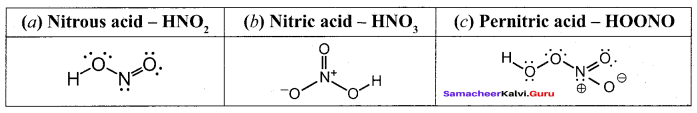Question 7.
Identify A, B and C from the following reactions.

1. P4 + Mg → A
2. P4+ Ca → B
3. P4 + Na → C

Answer:

1. P4 + 6Mg → 2Mg3P2 (Magnesium phosphide)
2. P4 + 6Ca → 2Ca3P2 (Calcium phosphide)
3. P4+ 12Na → 2Na3P (Sodium phosphide)

Question 8.
How will you prepare phosphine and explain the purification of phosphine?
Answer:
Phosphine is prepared by action of sodium hydroxide with white phosphorous in an inert atmosphere of carbon dioxide or hydrogen.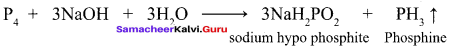Phosphine is freed from phosphine dihydride (P2H4) by passing through a freezing mixture. The dihydride condenses while phosphine does not. Phosphine can also prepared by the hydrolysis of metallic phosphides with water or dilute mineral acids.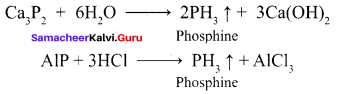Phosphine is prepared in pure form by heating phosphorous acid.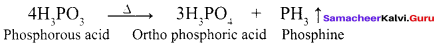A pure sample of phosphine is prepared by heating phosphonium iodide with caustic soda solution.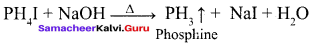Question 9.
What happens when PH3 reacts with oxygen or air?
Answer:
When phosphine air or oxygen it bums to give meta phosphoric acid.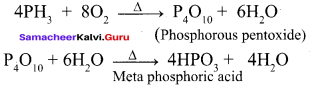Question 10.
Explain the structure of phosphine.
Answer:
In phosphine, phosphorus shows sp3 hybridisation. Three orbitals are occupied by bond pair and fourth comer is occupied by lone pair of electrons. Hence, bond angle is reduced to 94°. Phosphine has a pyramidal shape.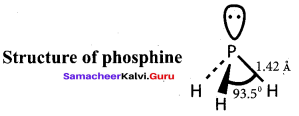Question 11.
Discuss the uses of phosphine.
Answer:
Phosphine is used for producing smoke screen as it gives large smoke. In a ship, a pierced container with a mixture of calcium carbide and calcium phosphide, liberates phosphine and acetylene when thrown into sea. The liberated phosphine catches fire and ignites acetylene. These burning gases serves as a signal to the approaching ships. This is known as Holmes signal.

Question 12.
How does PCl3 reacts with the following reagents?

1. C2H5OH
2. C2H5COOH

Answer:

1. C2H5OH + PCl3 → C2H5Cl + H3PO3
2. C2H5COOH + PCl3 → C2H5COCl + H3PO3

Question 13.
Explain the reaction between PCl5 and water.
Answer:
Phosphorous pentachloride reacts with water to give phosphoryl chloride and orthophosphoric acid.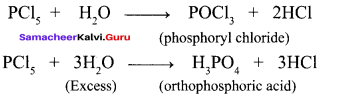Question 14.
Explain the structure of phosphorous trioxide (P2O3).
Answer:
In phosphorous trioxide four phosphorous atoms lie at the comers of a tetrahedron and six oxygen atoms along the edges. The P – O bond distance is 165.6 pm which is shorter than the single bond distance of P – O (184 pm) due to pπ – dπ bonding and results in considerable double bond character.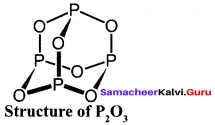Question 15.
Discuss the structure of phosphorous pentaoxide (P2O3).
Answer:
In P4O10 each P atoms form three bonds to oxygen atom and also an additional coordinate bond with an oxygen atom. Terminal coordinate P – O bond length is 143 pm, which is less than the expected single bond distance. This may be due to lateral overlap of filled p orbitals of an oxygen atom with empty d-orbital on phosphorous.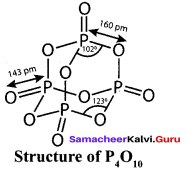Question 16.
Mention the uses of oxygen.
Answer:

1. Oxygen is one of the essential component for the survival of living organisms.
2. It is used in welding (oxyacetylene welding)
3. Liquid oxygen is used as fuel in rockets etc.

Question 17.
Give and explain reducing behaviour of sulphur dioxide.
Answer:
As Sulphur dioxide can readily be oxidised, it acts as a reducing agent. It reduces chlorine into hydrochloric acid.
SO2 + 2H2O + Cl2 → H2SO4 + 2HCl
It also reduces potassium permanganate and dichromate to Mn2+ and Cr3+ respectively.

• 2KMnO4 + 5SO2 + 2H2O → K2SO4 + 2MnSO4 + 2H2SO4
• K2Cr2O7 + 3SO2 + H2SO4 → K2SO4 + Cr2(SO4)3 + H2O

Question 18.
Why bleaching action of sulphur dioxide is temporary?
Answer:
In presence of water, sulphur dioxide bleaches coloured wool, silk, sponges and straw into colourless due to its reducing property.
SO2 + 2H2O → 2H2SO4 + 2(H)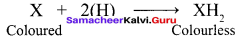However, the bleached product (colourless) is allowed to stand in air, it is reoxidised by atmospheric oxygen to its original colour. Hence bleaching action of sulphur dioxide is temporary.

Question 19.
Explain the structure of sulphur dioxide.
Answer:
In sulphur dioxide, sulphur atom undergoes sp2 hybridisation. A double bond arises between S and O is due to pπ- dπ overlapping.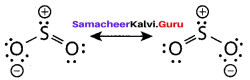Question 20.
How will you manufacture sulphuric acid by contact process?
Answer:
Manufacture of sulphuric acid by contact process involves the following steps:
1. Initially sulphur dioxide is produced by burning sulphur or iron pyrites in oxygen/air.
S + O2 → SO2
4FeS2 + 11O2 → 2Fe2O3 + 8SO2

2. Sulphur dioxide formed is oxidised to sulphur trioxide by air in the presence of a catalyst such as V2O5 or platinised asbestos.

3. The sulphur trioxide is absorbed in concentrated sulphuric acid and produces oleum (H2S2O7). The oleum is converted into sulphuric acid by diluting it with water.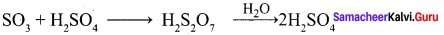To maximise the yield the plant is operated at 2 bar pressure and 720 K. The sulphuric acid obtained in this process is over 96 % pure.

Question 21.
Prove that H2SO4 is a strong dibasic acid.
Answer:
Sulphuric acid forms two types of salts namely sulphates and bisulphates.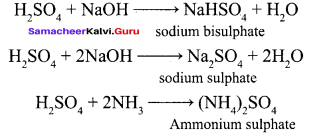Question 22.
Draw the structure of
(a) Marshall’s acid
(b) polythionic acid
(c) Dithionic acid
Answer: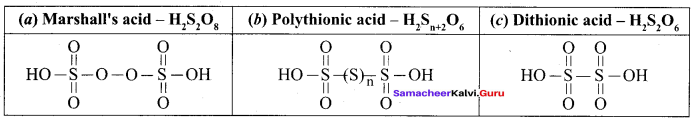Question 23.
What happens when chlorine reacts with ammonia?
Answer:
1. If chlorine reacts with excess ammonia, it gives nitrogen and ammonium chloride.
8NH3 + 3Cl2 → N2 + 6NH4Cl

2. If ammonia reacts with excess chlorine, it gives nitrogen trichloride and ammonium chloride.
4NH2 + 3Cl2 → NCl2 + 3NH4Cl

Question 24.
Bleaching action of chlorine is permanent. Justify this statement.
Answer:
Chlorine is a strong oxidising and bleaching agent because of the nascent oxygen.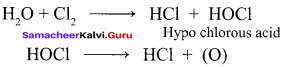Colouring matter + Nascent oxygen → Colourless oxidation product
Therefore, bleaching of chlorine is permanent. It oxidises ferrous salts to ferric, sulphites to sulphates and hydrogen sulphide to sulphur.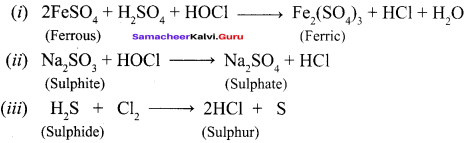Question 25.
Give the uses of chlorine.
Answer:

1. Purification of drinking water
2. Bleaching of cotton textiles, paper and rayon
3. It is used in extraction of gold and platinum

Question 26.
What is Royal water?
Answer:
When three parts of concentrated hydrochloric acid and one part of concentrated nitric acid are mixed, aquaregia is obtained. This is also known as Royal water. This is used for dissolving gold, platinum etc.
Au + 4H+ + $${ 4NO }_{ 3 }^{ – }$$ + 4 Cl → $${ AuCl }_{ 4 }^{ – }$$ + NO + 2H2O
3Pt + 16H+ + $${ 4NO }_{ 3 }^{ – }$$ + 18Cl → $${ 3PtCl }_{ 3 }^{ 2- }$$ + NO + 8H2O

Question 27.
Why HF is not stored in glass bottles?
Answer:
HF rapidly reacts with silica and glass to form soluble salt and that process leads to break the glass bottles. Hence HF is not stored in glass bottles.
SiO2 + 4HF → SiF4 + 2H2O
Na2SiO3 + 6HF → Na2SiF6 + 3H2O

Question 28.
Give the properties of inter halogen compounds.
Answer:
Properties of inter halogen compounds:

1. The central atom will be the larger one.
2. It can be formed only between two halogen and not more than two halogens.
3. Fluorine can’t act as a central metal atom being the smallest one.
4. Due to high electronegativity with small size fluorine helps the central atom to attain high coordination number
5. They can undergo the auto ionization.
6. They are strong oxidizing agents.

Question 29.
How will you prepare Xenon fluoride?
Answer:
Xenon fluorides are prepare by direct reaction of xenon and fluorine under different conditions as shown below.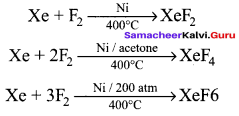Question 30.
Complete the following reaction.
(i) XeOF4 + SiO2 → A + SiF6
(ii) A + SiO2 → B + SiF6
Answer: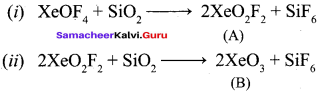Question 31.
What happens when XeF6 reacts 2.5 M solution of NaOH?
Answer:
When XeF6 reacts with 2.5 M of NaOH, sodium per xenate is obtained.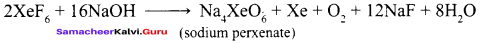Question 32.
Give two examples to show the anomalous behaviour of fluorine.
Answer:
The anomalous behaviour of fluorine is due to its:

1. Small size
2. highest electronegativity
3. low F-F bond dissociation enethalpy
4. non-availability of d-orbitals in its valence shell.

The two examples are:
1. Due to non-availability of d-orbitals in its valence shell, fluorine cannot expand its octet, therefore, shows only -1 oxidation state while all other halogens due to the presence of d-orbitals shows positive oxidation states of +1, +3, +5 and +7 besides oxidation state of -1.

2. Due to its small size, the three lone pair of electrons on each F atom in F – F molecule, repel the bond pair. As a result, F – F bond dissociation energy is lower than that of Cl – Cl bond.

Question 33.
Why does the reactivity of nitrogen differ from Phosphorus?
Answer:
Nitrogen exists as a diatomic molecule (N $$\equiv$$ N). Due to the presence of a triple bond between the two N – atoms the bond dissociation energy is large (941.4 kJ mol-1). As a result nitrogen is said to be chemically inert in its elemental state. In contrast, P – P single bond is much weaker (213 kJ mol-1) than N $$\equiv$$ N triple bond Therefore, phosphorus is much more reactive than nitrogen.

Question 34.
Why does NH3 form hydrogen bond but PH3 does not?
Answer:
The electronegativity of N (3.0) is much higher than that of FI (2.1). As a result, N – H bond is quite polar and hence NH3 undergoes intermolecular H-bonding. In contrast, both P and H have an electronegativity of 2.1. Therefore P – H bond is non-polar and hence PH3 does not undergo H – bonding.

Question 35.
Can PCl5 act as an oxidising as well as a reducing agent? Justify.
Answer:
Oxidation state of P in PCl5 is +5. Since P has five valence electrons in its valence shell, therefore it cannot increase its oxidation state beyond +5 by donating its electrons, therefore PCl5 cannot act as a reducing agent. However, it can decrease its oxidation number from +5 to +3 or some lower value, therefore PCl5 acts as an oxidising agent. For example, it oxidises Ag to AgCl.

Samacheer Kalvi 12th Chemistry p-Block Elements – II 5 Mark Questions and Answers

Question 1.
How does ammonia react with

1. Excess Cl2
2. Na
3. CuSO4
4. O2 / ∆

Answer:
1. Reaction with Excess Cl2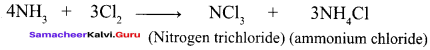2. Reaction with Na: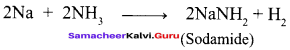3. Reaction with CuSO4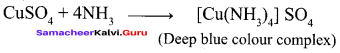4. Reaction with O2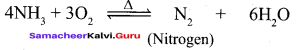Question 2.
Explain the reaction of metals with nitric acid.
Answer:
The reactions of metals with nitric acid are explained in 3 steps as follows:
Primary reaction:
Metal nitrate is formed with the release of nascent hydrogen
M + HNO3 → MNO3 + (H)

Secondary reaction:
Nascent hydrogen produces the reduction products of nitric acid.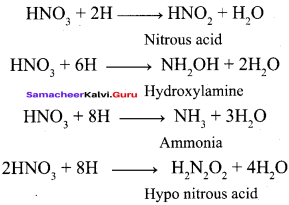Tertiary reaction:
The secondary products either decompose or react to give final products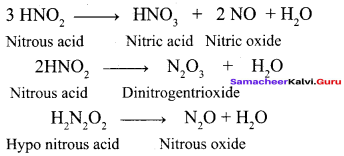For examples:
Copper reacts with nitric acid in the following manner
3Cu + 6HNO3 → 3Cu(NO3)2 + 6(H)
6(H) + 3HNO3 → 3HNO2 + 3H2O
3HNO2 → HNO3 + 2NO + H2O

Overall reaction
3Cu + 8HNO3 → 3CU(NO3)2 + 2NO + 4H2O

The concentrated acid has a tendency to form nitrogen dioxide
Cu + 4HNO3 → 3CU(NO3)2 + 2NO2 + 2H2O

Question 3.
How will you prepare ozone by laboratory method? Explain the structure of ozone.
Answer:
In the laboratory ozone is prepared by passing electrical discharge through oxygen. At a potential of 20,000 V about 10% of oxygen is converted into ozone it gives a mixture known as ozonised oxygen. Pure ozone is obtained as a pale blue gas by the fractional distillation of liquefied ozonised oxygen.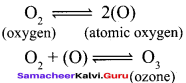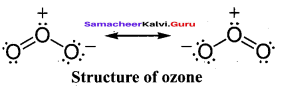Structure of ozone:
The ozone molecule have a bent shape and symmetrical with delocalised bonding between the oxygen atoms.

Question 4.
A is a king of acid. A reacts with HBr to give B and Bromine. A reacts with Na2CO3 to give C and carbon dioxide. Identify A, B and C. Give the reaction.
Answer:
1. King of acid is sulphuric acid (A).

2. Sulphuric acid (A) reacts with HBr to give SO2 (B) and Bromine.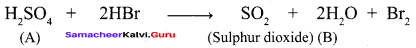3. Sulphuric acid (A) reacts with Na2CO3 to give sodium sulphate (C) and CO2.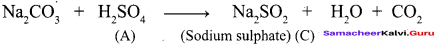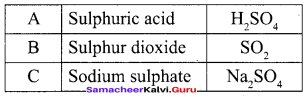Question 5.
How does Sulphuric acid react with the following:
(a) Al
(b) KNO3
(c) NaBr
(d) C6H6
Answer: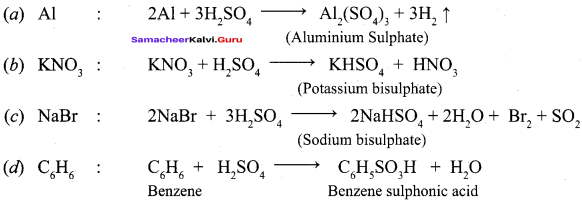Question 6.

1. Explain the test for sulphate (or) sulphuric acid.
2. What happens when sulphuric acid reacts with oxalic acid?

Answer:
1. Dilute solution of sulphuric acid or aqueous solution of sulphates gives white precipitate with barium chloride solution. It can also be detected using lead acetate solution. Here a white precipitate of lead sulphate is obtained.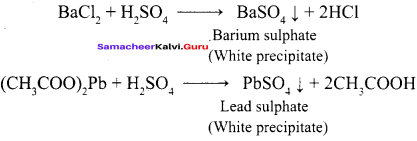2. Sulphuric acid reacts with oxalic acid to give CO and CO2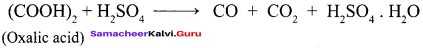Question 7.
Discuss the manufacture of chlorine.
Answer:
Electrolytic process:
When a solution of brine (NaCl) is electrolysed, Na+ and CP ions are formed. Na+ ion reacts with OH ions of water and forms sodium hydroxide. Hydrogen and chlorine are liberated as gases.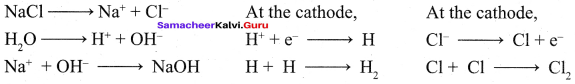Deacons process:
In this process a mixture of air and hydrochloric acid is passed up a chamber containing a number of shelves, pumice stones soaked in cuprous chloride are placed. Hot gases at about 723 K are passed through a jacket that surrounds the chamber.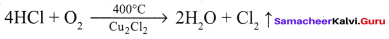The chlorine obtained by this method is dilute and is employed for the manufacture of bleaching powder. The catalysed reaction is given below,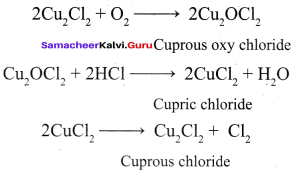Question 8.

1. How is bleaching powder prepared?
2. What happens when benzene reacts with chlorine?

Answer:
1. Bleaching powder is prepared by passing chlorine gas through dry slaked lime (Calcium hydroxide).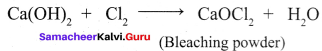2. Benzene reacts with chlorine in the presence of ferric chloride to give chlorobenzene.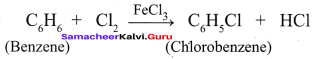Question 9.
Cone. H2SO4 is added followed by heating to each of the following test tubes labelled (I) to (IV). Identify in which of the above test tube the following change will be observed. Support your answer with the help of chemical equation:
(a) formation of black substance
(b) evolution of brown gas
(c) evolution of colourless gas
(d) formation of a brown substance which on dilution becomes blue.
(e) disappearance of yellow powder along with evolution of colourless gas.
Answer: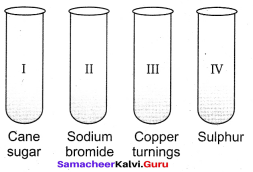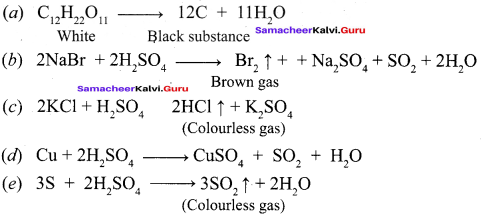Question 10.
Give reasons for each of the following:

1. Bleaching of flowers by Cl2 is permanent while by SO2 is temporary.
2. Molten aluminium bromide is a poor conductor of electricity.
3. Nitric oxide becomes brown when released in air.
4. PCl5 is ionic in nature in the solid state.
5. Ammonia is a good complexing agent.

Answer:
1. Cl2 bleaches by oxidation, while SO2 does it by reduction. The reduced product gets oxidise again and the colour is regained back.

2. Aluminium bromide exists as a dimer, Al2Br6. In this structure, each aluminium atom forms one coordinate bond by accepting a lone pair of electrons from the bromine atom of another aluminium bromide molecule and thus complete the octet of electrons. Due to lack of free electrons, molten aluminium bromide is a poor conductor of electricity.

3. Nitric oxide reacts with air and oxidised into NO2 which is brown in colour.
2NO + O2 → 2NO2

4. In solid state PCl5 exists as [PCl4]+ [PCl6] and hence it is ionic in nature. Due to its ionic nature, it conducts current on fusion.

5. N atom in ammonia has lone pair of electrons which can coordinate with other atoms or cations required for the stability of electron pair.

Question 11.
How will you prepare the following compounds.
(a) Hyponitrous acid
(b) Nitrous acid
(c) Pernitrous acid
(d) Pernitric acid
Answer: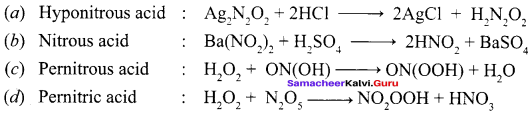Common Errors And Its Rectificaations:

Common Errors:

1. Oxidation number rules may be confused.
2. Oxidation number of oxygen may get confused.
3. Molecular formula and compound name may get confused.

Rectifications:

1. Oxidation number of fluorine is always -1.
2. Always oxygen is -2 but in peroxide it is -1. When it comes as a first element its oxidation number is positive..
3. Student should memorise the formula using short cut method, e.g. Phosphorous acid – H3PO3 Phosphoric acid – H3PO4 e.g. Phosphorous acid – H3PO3 By pronunciation, ‘O’ indicates oxygen is more.

Hope you love the Samacheer Kalvi 12th Chemistry Chapter Wise Material. Clearly understand the deep concept of Chemistry learning with the help of Tamilnadu State Board 12th Chemistry Chapter 3 p-Block Elements – II Questions and AnswersPDF. Refer your friends to and bookmark our website for instant updates. Also, keep in touch with us using the comment section.